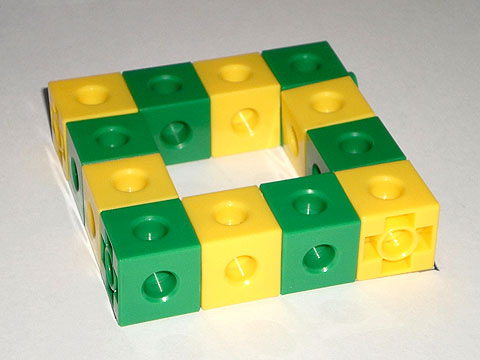Home > Patterns > Activities > Making border patterns

# Making border patterns

Making patterns around the border of a rectangle is a fun activity for young students, and it can teach them a lot about the unit of repeat.

Start by showing students this pattern, made from interlocking cubes.A border pattern.

After discussing how the pattern is made, give each student, or pair of students, 12 cubes (6 in one colour and 6 in another) and ask them to make a similar border pattern.

Now discuss the numbers.

• How many cubes are there altogether?
• How many are there of each colour?

Break the border into identical 'chunks'.

• How many pieces are there in each chunk?
• How many chunks are there?

This activity can be extended by giving students different-sized borders or different sets of cubes to make the border from. For example, you might ask them to make the same-sized border with 12 cubes in 3 different colours.

For more advanced students, pose hypothetical questions such as, "Is it possible to make a repeating pattern in a 3 $$\times$$ 4 border with a unit of repeat consisting of 3 cubes?"